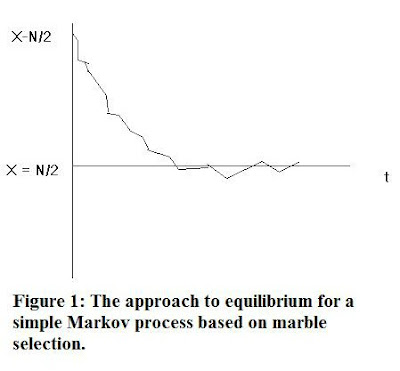## Friday, August 27, 2010

### Basic Statistical Mechanics of Order & DisorderIssues of order and disorder occupy the top rungs of that branch of physics we call statistical mechanics. In this discipline, we apply principles from classical mechanics as well as microscopic thermodynamics to arrive at properties of complex systems and their evolution. In so doing, we can expose the conditions under which spontaneous order can arise from chaos, as well as ascertain systems in which equilibrium applies.

One example isthe so-called Markov process which is at the heart of an important model used in statistical mechanics. As an example of this (Ehrenfest) model, one can consider a number of marbles, N, distributed among containers C1 and C2. At precise periods, say every 2 seconds, one marble is randomly selected from C1 (with X initial marbles at time to) and moved to C2 which must have N – X marbles by inference. It can be shown that at some arbitrary future time:

t(o) (less than or equal to) t Iess than or equal to) t(o) + tN/2

There will be either X + 1 or X –1 marbles, with transition probabilities: X/N corresponding to X -> X - 1, and 1 – X/N for X -> X + 1. Eventually, assuming the initial number of marbles large, equalization (‘equilibrium’) will occur with N/2 marbles in each container. The sketch of the approach to equilibrium is shown in Fig. 1.

We expect that as the process continues, oscillations will stabilize about the line X = N/2, so effectively the transition probabilities equalize. The important point of this process is that each such transition is independent of the system’s prior history. Say, there are just 16 marbles in all initially with 11 in C1 and 5 in C2 at t(o). Then successive distributions might appear like this, leading toward equilibrium (equalization of C1-C2 distributions):

C1: (11) -> (10) -> (9) ->(7) -> (9) -> (7) -> (9)

C2: (5) -> (6) -> (7) -> (9) -> (7) ->(9) ->(7)

Notice how the numbers relative to each container ultimately settle into small deviations from the equilibrium value (N/2 = 8). Of course, the probabilities reflect this, and can be represented on a separate graph for probability density plotted against X. This is shown in Fig. 2. In effect, this is the standard ‘Gaussian’ distribution. Mathematically, one can obtain it starting with the basic diffusion equation, @r/@t = D*DIV^2(rho) - where @ denotes partial derivative - and applying suitable boundary conditions, then using a Fourier transform to solve. This indicates all Markov processes can reduce to a form of diffusion.

The probability density peak at N/2 discloses this to be the most probable state. The reader should again bear in mind, however, that we are assuming thousands of marbles, transitions, to get it! Fig. 2 illustrates the direction in which all physical processes tend to run: from more order to more disorder. By definition, in physics the equilibrium (or ‘equalized’ distribution) state is the one with the greatest disorder.

An interesting analog to the above is based on polymers, using Monte Carlo simulations in what is called ‘the two space algorithm’. In one such computation it is found that a characteristic scale size for the polymer (given by a defined ‘radius of gyration’) fluctuates about some final equilibrium value. Such simulations would seem to have direct bearing on work that provides a model for pre-biotic evolution. In particular, that in given environments a single macromolecule can emerge to dominate, with all others fluctuations only.

What about processes that more clearly give rise to order out of chaos? Is such a thing possible? To address this, one needs to venture outside the domain of equilibrium processes, and delve into non-equilibrium ones. Indeed, these are observed in the cosmos at large. Thus, the formation of large scale structures like galaxies, galaxy clusters, gaseous nebulae and even superclusters can arise entirely from chaotic processes! One interesting model for the origin of all the matter in the universe is based upon a quantum fluctuation in its earliest epoch, engendering a primitive superdense radiation state followed by the non-equilibrium production of matter.

Next: Examining a simple spin-system model.

 This, of course, is from a thermodynamic viewpoint. In order to elicit thermodynamics from Markov processes, one must somehow assure that the probabilities in question approach that for the Boltzmann distribution, viz. P = exp(-E/kT)/Z, where Z is the partition function.

 See, e.g. Yaneer Bar-Yam, 1997: Dynamics of Complex Systems, Addison-Wesley, p. 499.

 See, e.g. Eigen, M. and Schuster, P.:1979, The Hypercycle, Springer-Verlag.

 The magnitude of a fluctuation can be obtained from probability parameters, in this case multiplying the rms deviation [N]^1/2/2) by 1/N, or in the case of the polymers, [R_G]^1/2 /2) by 1/[R_G]^1/2.

 Padmanabhan, T.: 1982, Universe Before Planck Time: A Quantum Gravity Model, in Physical Review D, Vol. 28, No. 4 (15 August, 1983), p. 756